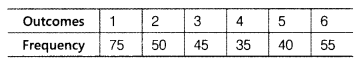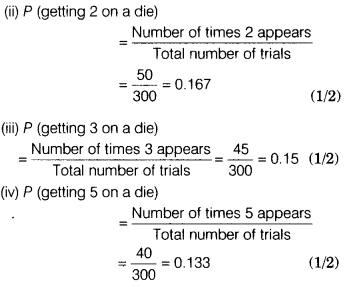# A die is thrown 300 times and the outcomes are noted as given below

A die is thrown 300 times and the outcomes are noted as given belowIf a die is thrown at random, then find the probability of getting prime number
(i) 1 (ii) 2 (iii) 3 (iv) 5

Here, total number of trials = 300
(i) We know that, 1 is not a prime number, so we cannot determine the probability of prime number.